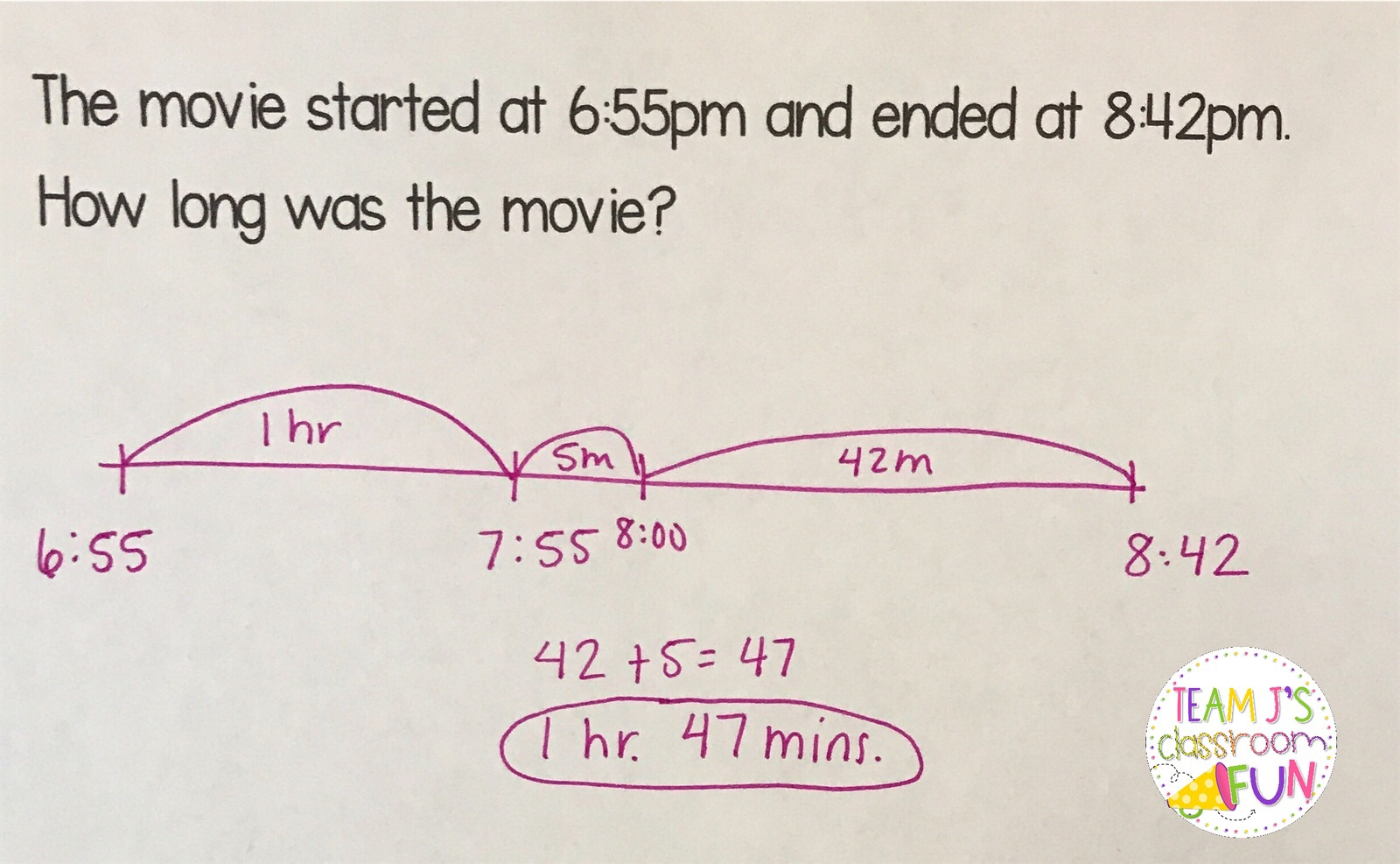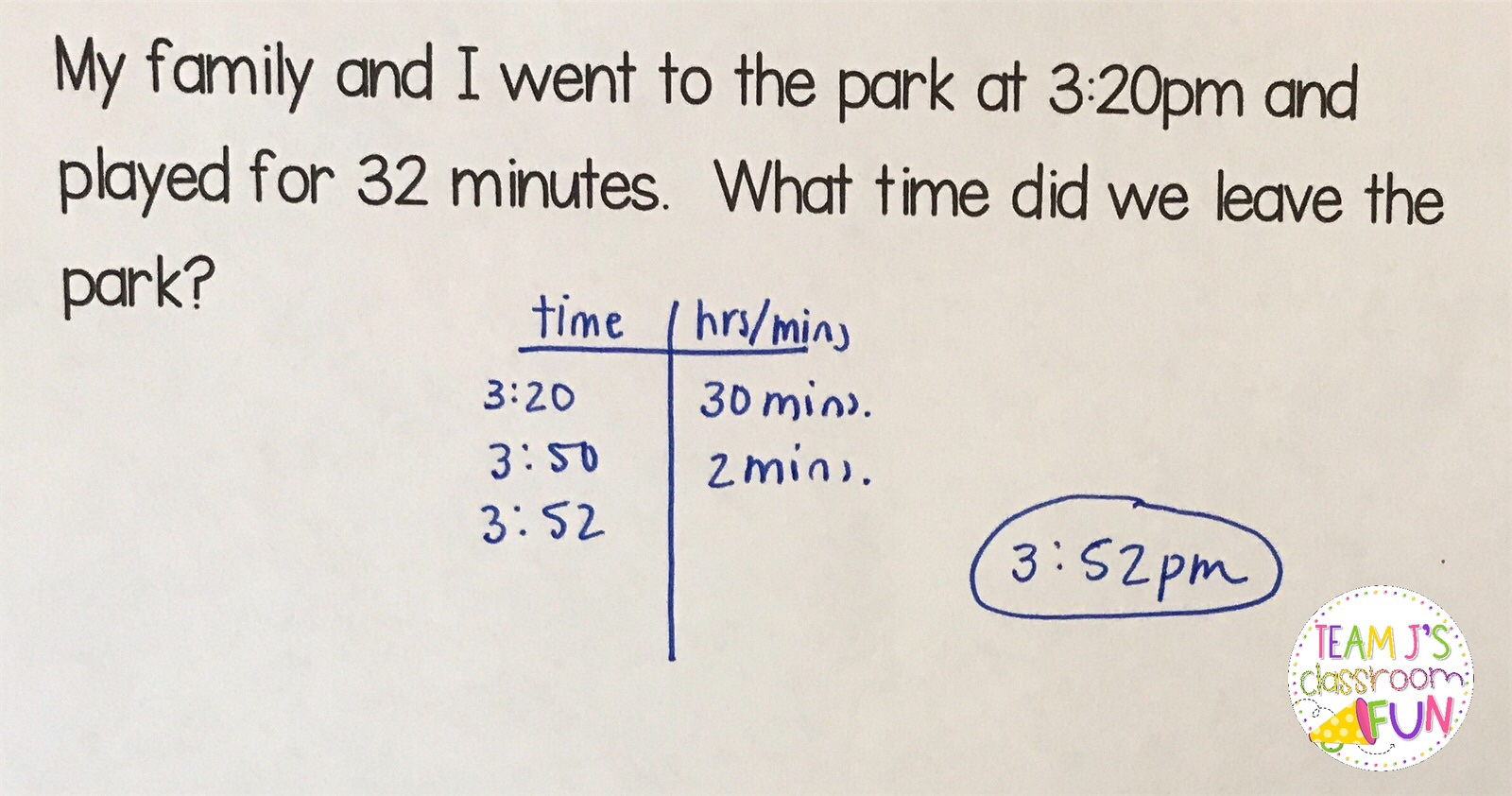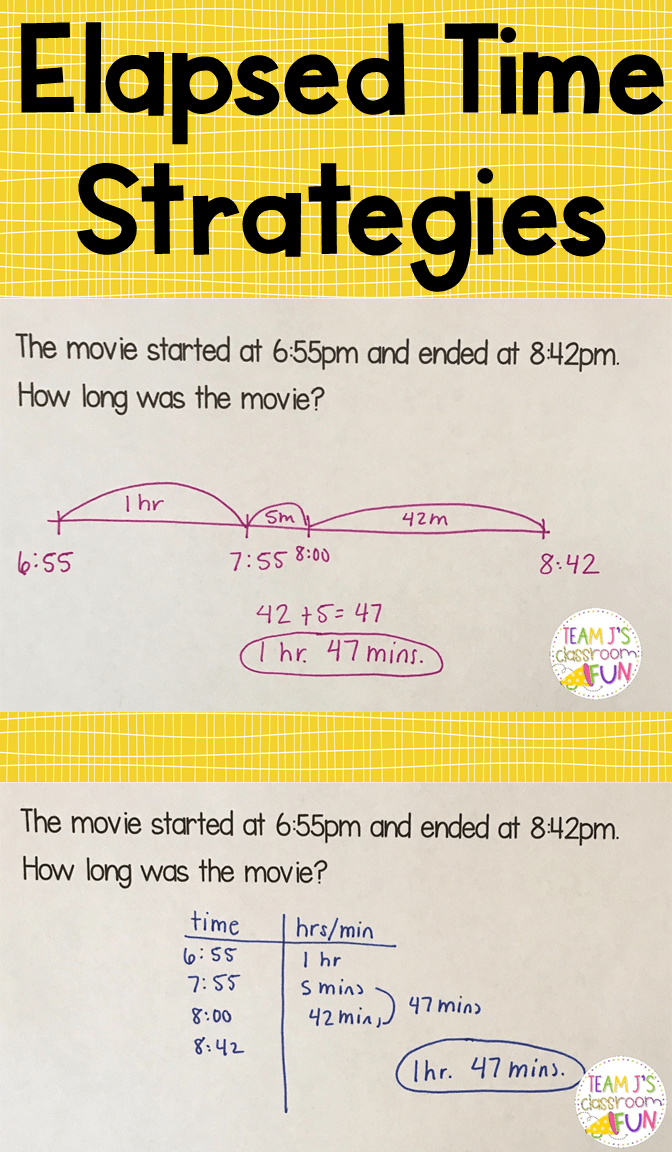Elapsed time can be a tricky skill for students to learn! But, with the right strategies they’ll understand it and be able to master it in no time!  Today I’m going to share my two favorite strategies for solving elapsed time problems.  These are elapsed time strategies that my second graders loved and helped them understand and easily solve these problems.  Now, I know the two strategies are very similar but they are laid out differently.  Some of my kids preferred the empty number line visual and some preferred the T-chart.

## Quick Tip

When working with elapsed time I like to use word problems.  I know you can give problems with a start and end time or a start time and elapsed time, but I like to use word problems. I find giving it in a story problem format helps kids visualize the problem because there is context.  This then makes the problem easier for them to solve.

## Strategy #1 – Empty Number LineFor empty number line, students start with an empty number line. Then, they place the start time at the beginning and end time at the end.  Next, students need to make jumps on the number line to figure out how much time has passed.  I suggest students always start by looking at hours since it is a larger chunk of time and then moving to minutes. Students may make jumps in different ways. For the problem below, some students might add five minutes to get to 8:00 and then make the jump of 42 minutes.  Other students may make jumps in increments of ten or twenty to get to the final time.  There is no “one” right way and what I like about this is students can make jumps that they are comfortable with given their math understanding. Once they have made their jumps they add the hours and minutes together to come up with the total elapsed time.This problem has the end time missing.  Students create the empty number line and put the start time on it.  Then using the elapsed time given – 32 minutes – they make jumps to find the end time.  Students can jump 30 minutes and then 2 minutes. Students can jump 10 minutes, 10 minutes, 10 minutes, and 2 minutes.  Students could also jump 20 minutes, 10 minutes, and 2 minutes.  Again the number line provides a visual strategy and then students can make jumps depending on their number flexibility and math foundation.

## Strategy #2 – T-ChartThe T-Chart strategy is very similar to the number line, but a different way to lay it out.  This strategy works better for some students.  To start, create a T-chart and write time on one side and hours/minutes on the other side.  In this example, the start time is written below the time side.  Then, students add time to the start time to get to the end time.  Students can start with hours or minutes, but I always suggest hours since it is a larger chunk of time.  So in this example, the student added an hour and got to 7:55.  Then, they added 5 minutes to get to 8:00. Next, they added the 42 minutes to get to 8:42.  Then they added the hour and minutes together to get the total answer. Again like the elapsed time strategy students can add the times in different increments.In this problem, the T-Chart is set up the same way as above.  The start time is written down and then the student adds the elapsed time given in the problem to find the end time.   The increments can be added in different ways (30 minutes, 10 minutes three times, 20 minutes and 10 minutes, etc).  Once the elapsed time is added the student will arrive at the end time, which is the answer.

Now there are more strategies than those two, but my students in the past have gravitated towards these two and found them to be the most helpful when solving these problems.  These two problem types are common, but the start time could also be unknown. In that case, students can use the same strategies and work backwards to find the beginning time.

Here is an anchor chart I created with my students when learning about elapsed time.## Resources

If you are interested in providing your students with some elapsed time word problem practice, check out my two resources below.

Elapsed Time Worksheets

These worksheets are easy for teachers to use because they are already differentiated!  There are three different levels for each worksheet. I’ve used these as homework, practice, and formative assessments.Elapsed Time Task Cards

Task cards are a great addition to math stations, math centers, or math practice time.  These are differentiated with three different sets!  I love using them because you’re able to meet the needs of all of your learners and they are able to all practice the same skill, but at their level.Previous Story
Next Story